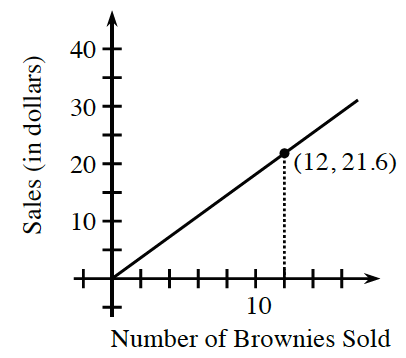### Home > CC3 > Chapter Ch6 > Lesson 6.2.2 > Problem6-59

6-59.Lashayia is famous for her delicious brownies, which she sells at football games. The graph at right shows the relationship between the number of brownies she sells and the amount of money she earns.

1. How much should she charge for 10 brownies? Be sure to demonstrate your reasoning.

Find the equation for this line.

Once you find the equation, substitute $10$ for $x$.

$y=\frac{21.6}{12}x$

$y=\frac{21.6}{12}\cdot10$

$y=18$ dollars

2. During the last football game, Lashayia made $\34.20$. How many brownies did she sell? Show your work.

Use the equation found in part (a) and set it equal to $\34.20$. Then solve for $x$.